# PostgreSQL SUBSTRING() with Example

## What is PostgreSQL Substring?

The PostgreSQL substring function helps you to extract and return part of a string. Instead of returning the whole string, it only returns a part of it.

In this PostgreSQL tutorial, you will learn:

## Syntax

The PostgreSQL substring function takes the following syntax:

```substring( string [from starting_position] [for length] )
```

## Parameters

 Name Description string The source string whose data type is varchar, char, string, etc. starting_position It is an optional parameter. It denotes the place where the extraction of the string will begin. If you omit this parameter, the extraction will start from position 1, which is the first character in the string. length It is an optional parameter. It denotes the number of characters to be extracted from the string. If you omit this parameter, the function will extract from starting_position to the end of the string.

## Examples

In this example, we want to extract the first 4 characters from the word Guru99:

```SELECT substring('Guru99' for 4);
```

The command will return the following: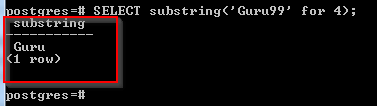We did not specify the starting position, so the extraction of the substring start at position 1. 4 characters were extracted to return the above.

The following example shows how to specify the starting position:

```SELECT substring('Guru99' from 1 for 4);
```

The command will return the following: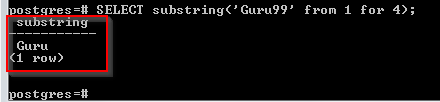We specified that the extraction of the substring should begin from position 1, and 4 characters should be extracted.

Let us extract 99 from the string Guru99:

```SELECT substring('Guru99' from 5);
```

The command will return the following: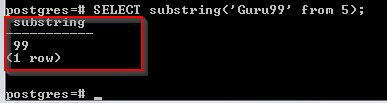We specified the starting position as 5. Since the number of characters to be extracted was not specified, the extraction ran to the end of the string.

Here is another example:

```SELECT substring('Guru99' from 5 for 2);
```

The command will return the following: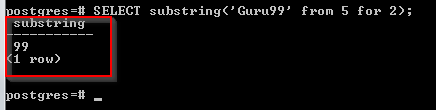We have started extraction at position 5, and 2 characters have been extracted.

Consider the Book table given below: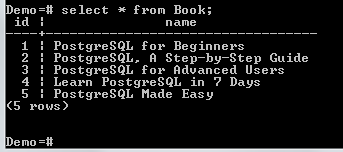We want to get a rough idea about the name of each book. However, we can extract only the first 15 characters from the name column of the table:

```SELECT
id,
SUBSTRING(name, 1, 15 ) AS name_initial
FROM
Book
ORDER BY
id;
```

The command will return the following:We now have a rough idea about the name of every book.

## Matching Substrings with SQL Regular Expression

In PostgreSQL, we can extract a substring matching a specified POSIX regular expression. In this case, the substring function is used with the following syntax:

```SUBSTRING(string FROM matching_pattern)
```

or

```SUBSTRING(string, matching_pattern);
```

Here is an explanation of the above parameters:

The string is the source string whose data type is varchar, char, string, etc.

The matching_pattern is the pattern to be used for searching in the string.

### Examples:

```SELECT
SUBSTRING (
'([0-9]{1,2})'
) as age;
```

The command will return the following: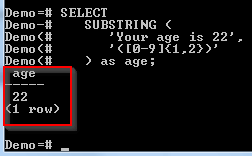Our input string is your age is 22. In the pattern, we are searching for a numeric pattern in our string when this is found, the substring function should only extract two characters.

Now let’s see how the actions are performed using pgAdmin.

The above queries where we don’t need a database can be executed directly from the query editor window. Just do the following:

Step 2) Click the Query Tool icon.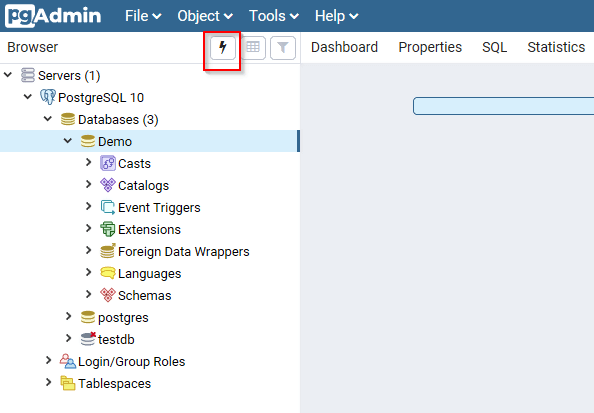The query editor window will be opened.

Step 3) Type the following query on the editor window.

```SELECT substring('Guru99' for 4);
```

Step 4) Click the Execute icon to execute the query.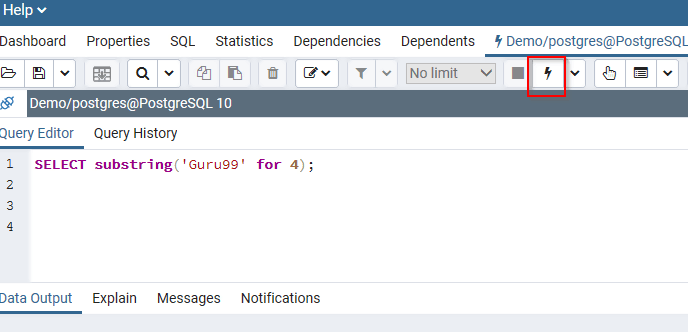It should return the following: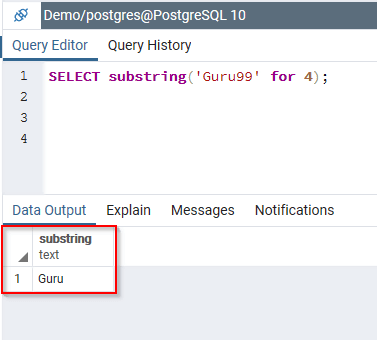Example 2:

```SELECT substring('Guru99' from 1 for 4);
```

It should return the following:Here is the next example:

```SELECT substring('Guru99' from 5);
```

It should return the following: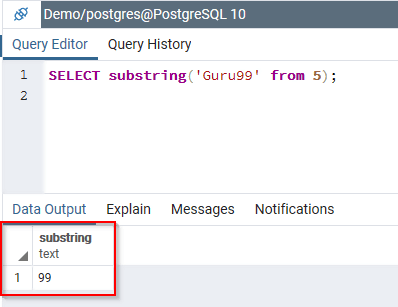Example 3:

```SELECT substring('Guru99' from 5 for 2);
```

It should return the following: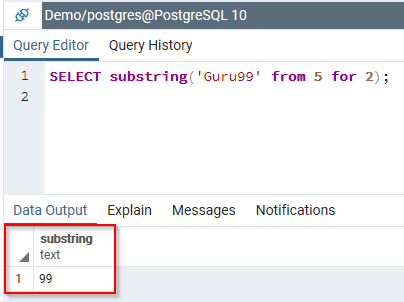Now, let us run the example using the Book table of the Demo database:

Step 2)

1. From the navigation bar on the left- Click Databases.
2. Click Demo.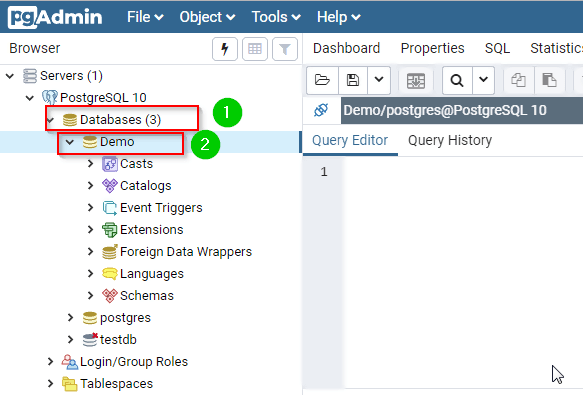Step 3) Type the query in the query editor:

```SELECT
id,
SUBSTRING(name, 1, 15 ) AS name_initial
FROM
Book
ORDER BY
id;
```

Step 4) Click the Execute button.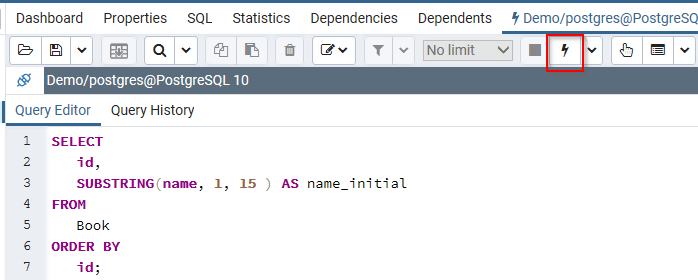It should return the following:We now have a basic idea of the name of every book.

### Matching Substrings with SQL Regular Expression

To accomplish the same on pgAdmin, do the following:

Step 2) Click the Query Tool icon.The query editor window will be opened.

Step 3) Type the following query on the editor window.

```SELECT
SUBSTRING (
'([0-9]{1,2})'
) as age;
```

Step 4) Click the Execute icon to execute the query.It should return the following:### Summary:

• The PostgreSQL Substring function helps in extracting and returning only a part of a string.
• The first character of the string is at position 1.
• If the number of characters to be extracted from the string is not specified, the function will extract characters from the specified start position to the end of the string.
• If the number of characters to be extracted is specified, only that number of characters will be extracted.